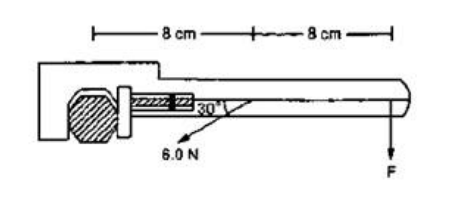# Solve the following :Question:

When a force of $6.0 \mathrm{~N}$ is exerted at $30^{\circ}$ to a wrench at a distance of $8 \mathrm{~cm}$ from the nut, it is just able to loosen the nut. What force F would be sufficient to loosen it if it acts perpendicularly to the wrench at $16 \mathrm{~cm}$ from the nut?Solution:

Torque in both the cases will be equal

$\tau_{1}=\tau_{2}$

$\left(6 \sin 30^{\circ}\right)(8)=(F)(16)$

$F=1.6 \mathrm{~N}$